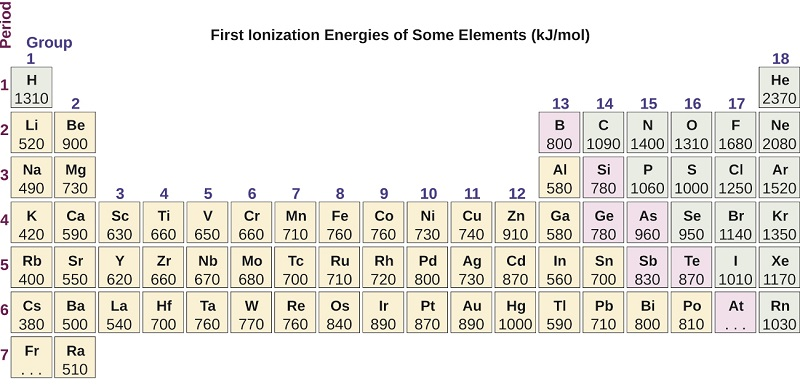# 3.2.2: Trends in Ionization Energy

$$\newcommand{\vecs}{\overset { \rightharpoonup} {\mathbf{#1}} }$$ $$\newcommand{\vecd}{\overset{-\!-\!\rightharpoonup}{\vphantom{a}\smash {#1}}}$$$$\newcommand{\id}{\mathrm{id}}$$ $$\newcommand{\Span}{\mathrm{span}}$$ $$\newcommand{\kernel}{\mathrm{null}\,}$$ $$\newcommand{\range}{\mathrm{range}\,}$$ $$\newcommand{\RealPart}{\mathrm{Re}}$$ $$\newcommand{\ImaginaryPart}{\mathrm{Im}}$$ $$\newcommand{\Argument}{\mathrm{Arg}}$$ $$\newcommand{\norm}{\| #1 \|}$$ $$\newcommand{\inner}{\langle #1, #2 \rangle}$$ $$\newcommand{\Span}{\mathrm{span}}$$ $$\newcommand{\id}{\mathrm{id}}$$ $$\newcommand{\Span}{\mathrm{span}}$$ $$\newcommand{\kernel}{\mathrm{null}\,}$$ $$\newcommand{\range}{\mathrm{range}\,}$$ $$\newcommand{\RealPart}{\mathrm{Re}}$$ $$\newcommand{\ImaginaryPart}{\mathrm{Im}}$$ $$\newcommand{\Argument}{\mathrm{Arg}}$$ $$\newcommand{\norm}{\| #1 \|}$$ $$\newcommand{\inner}{\langle #1, #2 \rangle}$$ $$\newcommand{\Span}{\mathrm{span}}$$$$\newcommand{\AA}{\unicode[.8,0]{x212B}}$$

Learning Objectives

• Describe and explain the observed trends in ionization energy of the elements

Video $$\PageIndex{1}$$: A brief overview of ionization energy.

#### Variation in Ionization Energies

The amount of energy required to remove the most loosely bound electron from a gaseous atom in its ground state is called its first ionization energy (IE1). The first ionization energy for an element, X, is the energy required to form a cation with +1 charge:

$\ce{X}(g)⟶\ce{X+}(g)+\ce{e-}\hspace{20px}\ce{IE_1}$

The energy required to remove the second most loosely bound electron is called the second ionization energy (IE2).

$\ce{X+}(g)⟶\ce{X^2+}(g)+\ce{e-}\hspace{20px}\ce{IE_2}$

The energy required to remove the third electron is the third ionization energy, and so on. Energy is always required to remove electrons from atoms or ions, so ionization processes are endothermic and IE values are always positive. For larger atoms, the most loosely bound electron is located farther from the nucleus and so is easier to remove. Thus, as size (atomic radius) increases, the ionization energy should decrease. Relating this logic to what we have just learned about radii, we would expect first ionization energies to decrease down a group and to increase across a period.Figure $$\PageIndex{1}$$: The first ionization energy of the elements in the first five periods are plotted against their atomic number.

Figure $$\PageIndex{1}$$ graphs the relationship between the first ionization energy and the atomic number of several elements. Within a period, the values of first ionization energy for the elements (IE1) generally increases with increasing Z. Down a group, the IE1 value generally decreases with increasing Z. There are some systematic deviations from this trend, however. Note that the ionization energy of boron (atomic number 5) is less than that of beryllium (atomic number 4) even though the nuclear charge of boron is greater by one proton. This can be explained because the energy of the subshells increases as l increases, due to penetration and shielding (as discussed previously in this chapter). Within any one shell, the s electrons are lower in energy than the p electrons. This means that an s electron is harder to remove from an atom than a p electron in the same shell. The electron removed during the ionization of beryllium ([He]2s2) is an s electron, whereas the electron removed during the ionization of boron ([He]2s22p1) is a p electron; this results in a lower first ionization energy for boron, even though its nuclear charge is greater by one proton. Thus, we see a small deviation from the predicted trend occurring each time a new subshell begins.Figure $$\PageIndex{2}$$: This version of the periodic table shows the first ionization energy of (IE: 1), in kJ/mol, of selected elements.

Another deviation occurs as orbitals become more than one-half filled. The first ionization energy for oxygen is slightly less than that for nitrogen, despite the trend in increasing IE1 values across a period. Looking at the orbital diagram of oxygen, we can see that removing one electron will eliminate the electron–electron repulsion caused by pairing the electrons in the 2p orbital and will result in a half-filled orbital (which is energetically favorable). Analogous changes occur in succeeding periods (note the dip for sulfur after phosphorus in Figure $$\PageIndex{2}$$.Removing an electron from a cation is more difficult than removing an electron from a neutral atom because of the greater electrostatic attraction to the cation. Likewise, removing an electron from a cation with a higher positive charge is more difficult than removing an electron from an ion with a lower charge. Thus, successive ionization energies for one element always increase. As seen in Table $$\PageIndex{1}$$, there is a large increase in the ionization energies (color change) for each element. This jump corresponds to removal of the core electrons, which are harder to remove than the valence electrons. For example, Sc and Ga both have three valence electrons, so the rapid increase in ionization energy occurs after the third ionization.

Table $$\PageIndex{1}$$: Successive Ionization Energies for Selected Elements (kJ/mol)
Element IE1 IE2 IE3 IE4 IE5 IE6 IE7
K 418.8 3051.8 4419.6 5876.9 7975.5 9590.6 11343
Ca 589.8 1145.4 4912.4 6490.6 8153.0 10495.7 12272.9
Sc 633.1 1235.0 2388.7 7090.6 8842.9 10679.0 13315.0
Ga 578.8 1979.4 2964.6 6180 8298.7 10873.9 13594.8
Ge 762.2 1537.5 3302.1 4410.6 9021.4 Not available Not available
As 944.5 1793.6 2735.5 4836.8 6042.9 12311.5 Not available

Example $$\PageIndex{1}$$: Ranking Ionization Energies

Predict the order of increasing energy for the following processes: IE1 for Al, IE1 for Tl, IE2 for Na, IE3 for Al.

Solution

Removing the 6p1 electron from Tl is easier than removing the 3p1 electron from Al because the higher n orbital is farther from the nucleus, so IE1(Tl) < IE1(Al). Ionizing the third electron from

$\ce{Al}\hspace{20px}\ce{(Al^2+⟶Al^3+ + e- )} \nonumber$

requires more energy because the cation Al2+ exerts a stronger pull on the electron than the neutral Al atom, so IE1(Al) < IE3(Al). The second ionization energy for sodium removes a core electron, which is a much higher energy process than removing valence electrons. Putting this all together, we obtain:

IE1(Tl) < IE1(Al) < IE3(Al) < IE2(Na).

Exercise $$\PageIndex{1}$$

Which has the lowest value for IE1: O, Po, Pb, or Ba?

Ba

## Summary

Video $$\PageIndex{2}$$: A brief review of ionization energy.

Ionization energy (the energy associated with forming a cation) decreases down a group and mostly increases across a period because it is easier to remove an electron from a larger, higher energy orbital.

### Glossary

ionization energy
energy required to remove an electron from a gaseous atom or ion. The associated number (e.g., second ionization energy) corresponds to the charge of the ion produced (X2+)

• Adelaide Clark, Oregon Institute of Technology
• Crash Course Chemistry: Crash Course is a division of Complexly and videos are free to stream for educational purposes.